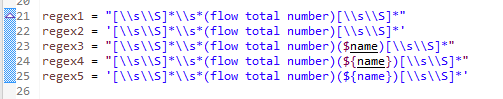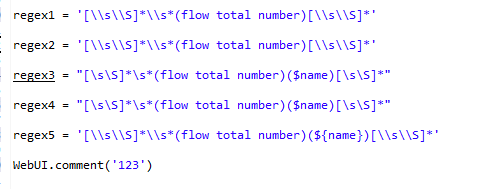Operation process

1. the definition value contains \$in double quotation marks, including \ \ two slashes

for example: regex = “[\s\s]\s (flow total number) (\${name}) [\s\s]*”

Operation results

\ \ in the value becomes , {} is deleted

for example: regex = “[\s\s]\s (flow total number) (\$name) [\s\s]*”

Expected results

Adding or adjusting the comment position will not change the parameter value1 Like

In Groovy language, string interpolation works only in a double quoted string.

``````def name = 'Alice'

assert "\${name}" == 'Alice'   // -> pass, the double-quoted string "\${name}" is interpolated to 'Alice'
assert '\${name}' == 'Alice'   // -> fail, the single-quoted string '\${name}' stays unchanged
``````